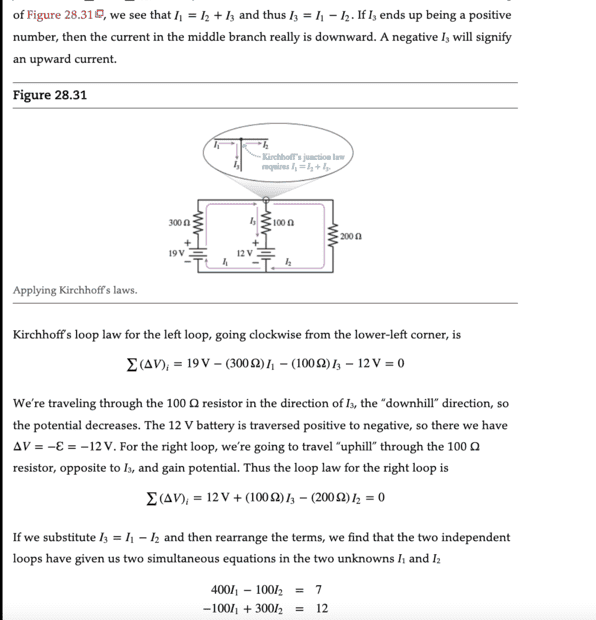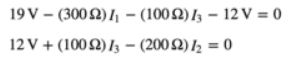# Trouble finding the current through this resistor in the circuit

• guyvsdcsniper

#### guyvsdcsniper

Homework Statement
FIind the current through and the potential difference across the 100 ohm resistor.
Relevant Equations
junction rule. loop rule
I was following this problem up until they say we can they state I3= I1-I2. I understand why we can say that but I don't see how I can use that to get the system of equation in terms of I1 and I2 at the bottom. Could someone show me how this was done?You have the two equationsTake the first of these two equations and substitute ##I_3 = I_1-I_2##. This means to replace ##I_3## in the equation by the quantity ##(I_1-I_2)##. Then simplify.

Do the same for the second equation.

Show your work so we can help with any particular step for which you have trouble.

•guyvsdcsniper, Steve4Physics, DaveE and 1 other person